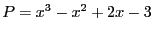Next: Laguerre method Up: Lagrange-MacLaurin theorem Previous: Implementation   Contents

Example

Letand the procedure:

Coeff(1)= -3;Coeff(2)=2;Coeff(3)=-1;Coeff(4)=1;
Num=MacLaurin_Bound_Interval(3,Coeff,&bound);
Coeff_App(1)= INTERVAL(-3.1,-2.9);Coeff_App(2)=INTERVAL(1.9,2.1);
Coeff_App(3)=INTERVAL(-1.1,-0.9);Coeff_App(4)=INTERVAL(0.9,1.1);
Num=MacLaurin_Bound_Interval(3,Coeff_App,Bound);
In the first case we find that all the roots are lower than 4 and in the second that the roots have bounds [4.44444,4.44444]. If we have used the lower bound procedures we will have found that all the roots are greater than -1.

Jean-Pierre Merlet 2012-12-20